# Apparently ambiguous times

My spring semester schedule is such that on certain days I have a four-hour break between my classes, which is an ideal time to get research done, although in practice most of that time is wasted on the web.   The other day I glanced up at my wall clock to gauge the time, and was surprised when I saw this: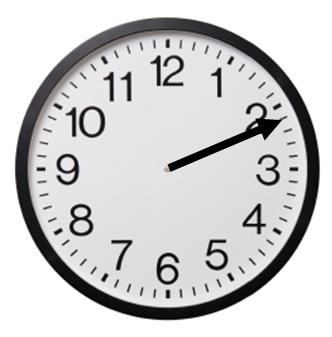I first I though the hour hand had fallen off the clock (which had happened to a wristwatch I owned) and was momentarily panicked at the prospect of missing my afternoon class (or rather, being 11 minutes late to it). However, a few seconds later the minute hand moved a smidgen more to reveal that it had simply obscured the hour hand entirely, indicating a time of 2:11.

That got me to thinking… What are the other such “apparently ambiguous times,” times at which the hour hand and the minute hand both point in the same direction? Generalizing a bit, what are the “really apparently ambiguous times” at which the hour, minute, and second hands all point in the same direction?

It’s a little fun to figure out, and I won’t deprive you of it until after the jump.

Let’s deal with the hour and minute hand question first. Let$h(t)$ and$m(t)$ denote the clockwise angles (in degrees) the hour-hand and the minute-hand, respectively, make with “high noon” at hour$t$. In particular, the minute hand completes a full revolution in one hour, so$m(t) = 360 cdot t$,

while the hour hand only makes one-twelfth a revolution, so$displaystyle h(t) = 360 cdot frac{t}{12} = 30 cdot t$.

The two hands will point in the same direction whenever there respective angle functions differ by a multiple of 360, so we need only solve the equation$m(t) = h(t) + 360 k$. Solving this yields$displaystyle t = frac{12}{11} k$,

which means the two hands point in the same direction every$1 frac{1}{11}$ hours. Note that one-eleventh of an hour is$5 frac{5}{11}$ minutes, or five minutes and$27 frac{3}{11}$ seconds.

Hence, starting from 12 o’clock, the two hands will realign every 1 hour, 5 minutes, and 27.2727… seconds, doing this a grand total of eleven times before reaching 12 o’clock again. To the nearest second, these times are:

12:00:001:05:27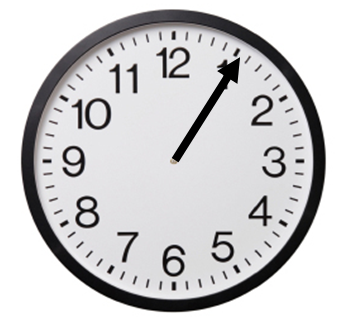2:10:553:16:22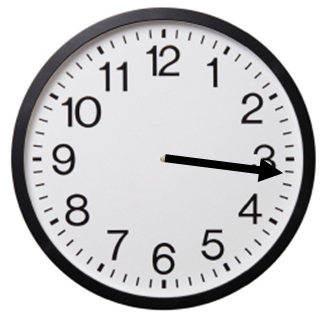4:21:49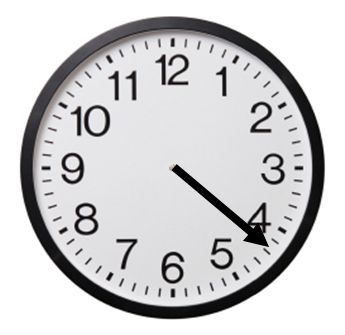5:27:16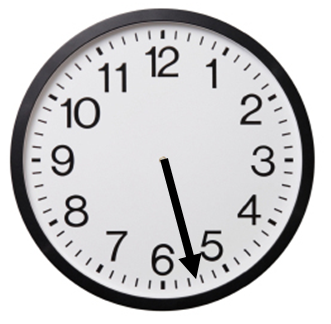6:32:44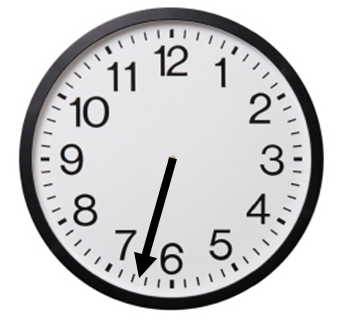7:38:11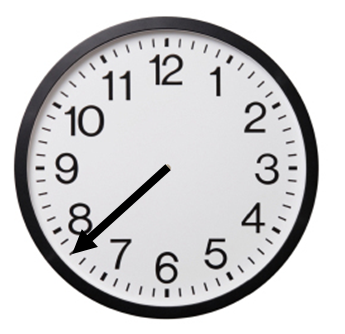8:43:38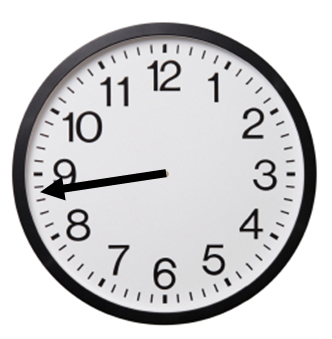9:49:05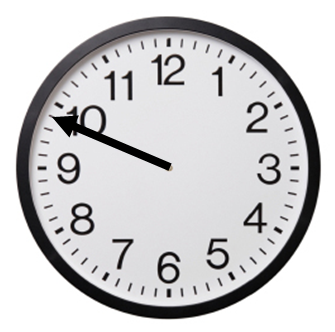10:54:33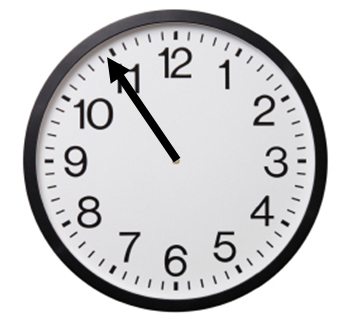How about the issue of all three hands (hour, minute, second)   in agreement?

Well, given the solution above, this is easy.   If all three hands agree, then the first two agree, so it must be at one of the 11 times indicated above.   It’s pretty easy to now sketch the appropriate second hand to determine that it’s only at high noon or the stroke of midnight that this event occurs.

This entry was posted in mathify. Bookmark the permalink.